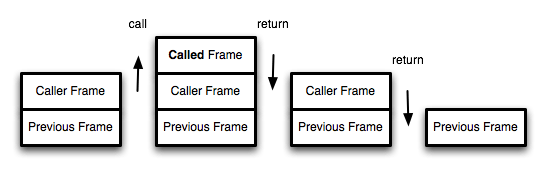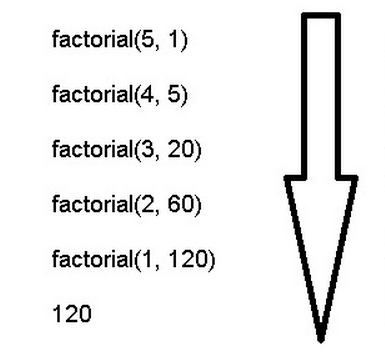# Tail call optimization

Last call (Tail Call) is an important concept in functional programming, this article describes its meaning and usage.## 1. What is a tail call?

The concept of tail call is very simple. It can be said clearly in one sentence, which means that the last step of a function is to call another function.

``````
function f(x){
return g(x);
}
``````

In the above code, the last step of function f is to call function g, which is called tail call.

The following two cases are not tail calls.

``````
// 情况一
function f(x){
let y = g(x);
return y;
}

// 情况二
function f(x){
return g(x) + 1;
}
``````

In the above code, the first situation is that there are other operations after the function g is called, so it is not a tail call, even if the semantics are exactly the same. Case 2 also belongs to the operation after the call, even if it is written in one line.

The tail call does not necessarily appear at the end of the function, as long as it is the last operation.

``````
function f(x) {
if (x > 0) {
return m(x)
}
return n(x);
}
``````

In the above code, functions m and n are both tail calls, because they are both the last operation of function f.

## Two, tail call optimization

The reason why the tail call is different from other calls lies in its special call location.

We know that a function call will form a “call record” in the memory, also known as a “call frame”, which saves information such as the call location and internal variables. If function B is called inside function A, then a call record of B will also be formed above the call record of A. Wait until B runs to the end, return the result to A, B’s call record will disappear. If function B also calls function C, there is also a call log stack of C, and so on. All call records form a “call stack” (call stack).Since the tail call is the last operation of the function, there is no need to keep the call record of the outer function, because the call location, internal variables and other information will not be used anymore, just use the call record of the inner function directly to replace the outer function The call record is fine.

``````
function f() {
let m = 1;
let n = 2;
return g(m + n);
}
f();

// 等同于
function f() {
return g(3);
}
f();

// 等同于
g(3);
``````

In the above code, if function g is not a tail call, function f needs to save the values ​​of internal variables m and n, the call location of g, and other information. But since the function f ends after g is called, it is possible to delete the call record of f() at the last step, and only keep the call record of g(3).

This is called “Tail call optimization”, that is, only the call records of inner functions are kept. If all functions are tail calls, then it is possible to achieve that there is only one call record each time it is executed, which will greatly save memory. This is the meaning of “tail call optimization”.

## Three, tail recursion

The function calls itself, which is called recursion. If the tail calls itself, it is called tail recursion.

Recursion is very memory intensive, because thousands or hundreds of call records need to be saved at the same time, and it is prone to “stack overflow” errors. But for tail recursion, because there is only one call record, the “stack overflow” error will never occur.

``````
function factorial(n) {
if (n === 1) return 1;
return n * factorial(n - 1);
}

factorial(5) // 120
``````

The above code is a factorial function. To calculate the factorial of n, at most n call records need to be saved, and the complexity is O(n).

If rewritten as tail recursion, only one call record is kept, and the complexity is O(1).

``````
function factorial(n, total) {
return factorial(n - 1, n * total);
}

factorial(5, 1) // 120
``````It can be seen that “tail call optimization” is of great significance to recursive operations, so some functional programming languages ​​have written it into the language specifications. The same is true for ES6. For the first time, it is clearly stated that all implementations of ECMAScript must deploy “tail call optimization”. This means that in ES6, as long as tail recursion is used, there will be no stack overflow, which saves memory.

## Fourth, the rewrite of the recursive function

The implementation of tail recursion often needs to rewrite the recursive function to ensure that the last step only calls itself. The way to do this is to rewrite all the internal variables used into function parameters. For example, in the above example, the factorial function factorial needs an intermediate variable total, so rewrite this intermediate variable as a function parameter. The disadvantage of this is that it is not intuitive, and it is difficult to see at first glance. Why do we need to pass in two parameters 5 and 1 to calculate the factorial of 5?

Two methods can solve this problem. The first method is to provide a normal form of function in addition to the tail recursive function.

``````
function tailFactorial(n, total) {
return tailFactorial(n - 1, n * total);
}

function factorial(n) {
return tailFactorial(n, 1);
}

factorial(5) // 120
``````

The above code uses a normal factorial function factorial to call the tail recursive function tailFactorial, which looks much more normal.

Functional programming a concept called curried (as currying), meaning that the transfer function parameters into a single multi-parameter form. Currying can also be used here.

``````
function currying(fn, n) {
return function (m) {
return fn.call(this, m, n);
};
}

function tailFactorial(n, total) {
return tailFactorial(n - 1, n * total);
}

const factorial = currying(tailFactorial, 1);

factorial(5) // 120
``````

The above code uses currying to change the tail recursive function tailFactorial into a factorial that only accepts 1 parameter.

The second method is much simpler, which is to use ES6 function default values.

``````
function factorial(n, total = 1) {
return factorial(n - 1, n * total);
}

factorial(5) // 120
``````

In the above code, the parameter total has a default value of 1, so there is no need to provide this value when calling.

To sum up, recursion is essentially a loop operation. Purely functional programming languages ​​do not have loop operation commands. All loops are implemented by recursion, which is why tail recursion is extremely important for these languages. For other languages ​​that support “tail call optimization” (such as Lua, ES6), you only need to know that loops can be replaced by recursion, and once recursion is used, it is best to use tail recursion.

## Five, strict mode

ES6 tail call optimization is only enabled in strict mode, and normal mode is invalid.

This is because in normal mode, there are two variables inside the function, which can track the call stack of the function.

• ``` arguments ``` : Returns the parameters of the function at the time of the call.
• ``` func.caller ``` : Returns the function that called the current function.

When tail call optimization occurs, the call stack of the function will be rewritten, so the above two variables will be distorted. Strict mode disables these two variables, so tail call mode only takes effect in strict mode.